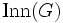Outer automorphism group

Contents

Let$G$ be a group. The outer automorphism group of$G$, denoted$\operatorname{Out}(G)$, is defined as the quotient group of the automorphism group$\operatorname{Aut}(G)$ by the inner automorphism group$\operatorname{Inn}(G)$.